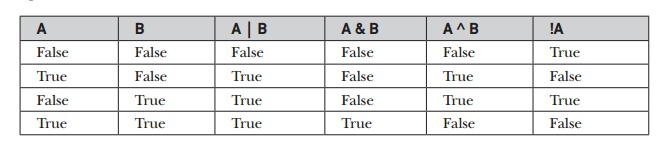Home | | Web Programming | Boolean Logical Operators - Java

# Boolean Logical Operators - Java

The Boolean logical operators shown here operate only on boolean operands. All of the binary logical operators combine two boolean values to form a resultant boolean value.

Boolean Logical Operators

The Boolean logical operators shown here operate only on boolean operands. All of the binary logical operators combine two boolean values to form a resultant boolean value.The logical Boolean operators, &, |, and ^, operate on boolean values in the same way that they operate on the bits of an integer. The logical ! operator inverts the Boolean state: !true == false and !false == true. The following table shows the effect of each logical operation:Here is a program that is almost the same as the BitLogic example shown earlier, but it operates on boolean logical values instead of binary bits:

// Demonstrate the boolean logical operators.

class BoolLogic {

public static void main(String args[]) {

boolean a = true;

boolean b = false;

boolean c = a | b;

boolean d = a & b;

boolean e = a ^ b;

boolean f = (!a & b) | (a & !b);

boolean g = !a;

System.out.println(" a = " + a);

System.out.println(" b = " + b);

System.out.println(" a|b = " + c);

System.out.println(" a&b = " + d);

System.out.println(" a^b = " + e);

System.out.println("!a&b|a&!b = " + f);

System.out.println(" !a = " + g);

}

}

After running this program, you will see that the same logical rules apply to boolean values as they did to bits. As you can see from the following output, the string representation of a Java boolean value is one of the literal values true or false:

a = true b = false

a|b = true a&b = false a^b = true

!a&b|a&!b = true !a = false

Short-Circuit Logical Operators

Java provides two interesting Boolean operators not found in some other computer languages. These are secondary versions of the Boolean AND and OR operators, and are commonly known as short-circuit logical operators. As you can see from the preceding table, the OR operator results in true when A is true, no matter what B is. Similarly, the AND operator results in false when A is false, no matter what B is. If you use the || and && forms, rather than the | and & forms of these operators, Java will not bother to evaluate the right-hand operand when the outcome of the expression can be determined by the left operand alone. This is very useful when the right-hand operand depends on the value of the left one in order to function properly. For example, the following code fragment shows how you can take advantage of short-circuit logical evaluation to be sure that a division operation will be valid before evaluating it:

if (denom != 0 && num / denom > 10)

Since the short-circuit form of AND (&&) is used, there is no risk of causing a run-time exception when denom is zero. If this line of code were written using the single & version of AND, both sides would be evaluated, causing a run-time exception when denom is zero.

It is standard practice to use the short-circuit forms of AND and OR in cases involving Boolean logic, leaving the single-character versions exclusively for bitwise operations. However, there are exceptions to this rule. For example, consider the following statement:

if(c==1 & e++ < 100) d = 100;

Here, using a single & ensures that the increment operation will be applied to e whether c is equal to 1 or not.

Study Material, Lecturing Notes, Assignment, Reference, Wiki description explanation, brief detail
Java The Complete Reference : The Java Language : Operators : Boolean Logical Operators - Java |